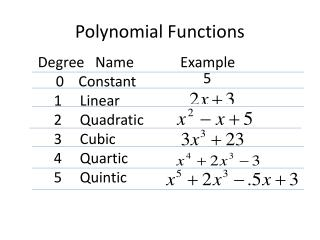DownloadDownload PresentationPolynomial Functions

# Polynomial Functions

Télécharger la présentation## Polynomial Functions

- - - - - - - - - - - - - - - - - - - - - - - - - - - E N D - - - - - - - - - - - - - - - - - - - - - - - - - - -
##### Presentation Transcript

1. Degree Name Example • 0 Constant • Linear • Quadratic • Cubic • Quartic • Quintic 5 Polynomial Functions

2. - + 2 x 4x 3=0 2nd Degree Polynomials and the Zeros of the Function Factor the polynomial and find the points At which the function equals zero. 3 can only be factored by 1 and 3. 1 + 3 = 4, so we can factor by inspection getting: So the zeros (roots) of the equation are 1,3.

3. + + 2 ax bx c=0 2nd Degree Polynomials and the Zeros of the Function Another way to factor is to use the quadratic equation. Put the equation in standard form: Insert the coefficients into the quadratic equation as follows:

4. 2nd Degree Polynomials and the Zeros of the Function Let’s use the quadratic to solve a 2nd degree equation: = 0 Insert the coefficients into the quadratic equation.

5. 2nd degree polynomials and the zeros of the function so x = 1, -4 so The roots (zeros) of the equation are the 1 and -4. In a 2nd degree polynomial there will be 2 roots.

6. Working with Cubic and Quartic Polynomial equations Find the roots of this cubic equation using synthetic division: Let’s try x = -1: Zero for the Remainder shows that x=-1 is a root. This will be the equation remaining: Now with the 2nd degree polynomial left, roots can be Found using factoring, or the quadratic equation.

7. Working with Cubic and Quartic Polynomial Equations Find the roots of the following quartic equation: Start with x=1 and use synthetic division: x = 1 is a root, and the remaining equation is:

8. Working with Cubic and Quartic Polynomial Equations Using factoring by grouping on our remaining equation: We see that: And our remaining roots are:

9. Use of synthetic substitution Using synthetic substitution to find the value of f(x) we proceed as follows: For example: The answer is the remainder shown here:

10. Applications of quadratic equations Quadratic equations can be used for finding maximum And minimum values for various items, as shown in the Following example. A rectangular yard is built using a wall for one side, and using 60 feet of fencing for the 3 other sides of the yard. What dimensions will give the greatest area for the yard? 60-2x x x Wall Our equation for the area of the yard will be:

11. Applications of Quadratic Equations So solving the equation (from the last page) we get: To find the maximum value for x we use: So using x = 15, we see that the yard’s dimensions will be 15 x 30, with a maximum area of 450 square feet.